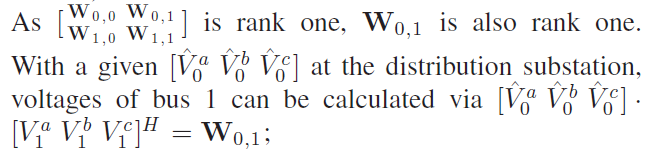# How to calculate unknown vectors from semidefinite square matrix variable

Hello All,

I have a question regarding how to calculate unknown vector from sdp matrix.
I have a known vector of X=[X1 X2 X3], where X1, X2 and X3 are complex numbers and known, and I also have a vector of Y=[Y1 Y2 Y3].
I defined my sdp matrix of W = X*Y’, my question is how can i get Y vector variables using W matrix?

Any idea?

Thanks

Can you please state more clearly what is known, i.e., what are the inputs and constraints, and what is to be found? Do you have a well-thought through mathematical problem?

Note that if X and Y are column vectors, then `X*Y' i`s a rank one matrix, i.e., all but one eigenvalues = 0.

As I understand your problem, you have a rank constraint (if your decision variable is indeed W) which is a non convex constraint.

Yes, rank(W) should be 1, but I do not use it under constraints; my only constraint on W is W >= 0.
Actually what I’m trying to do is explained as following:How can I get [V1^a V1^b V1^c]’ vector?

Thanks,

Please provide a self-contained and complete mathematical description of the problem, with a consistent notation. Exactly what the inputs are, what is known, what the constraints are, and what is unknown.

As currently written, mind-reading ability seems to be needed more so than optimization or linear algebra knowledge in order to solve your problem, whatever it is.

Mark’s actually being more patient that I am (thank you Mark!)

This forum is not for general modeling assistance, it is for assistance on actual CVX usage. You’ve presented no code here so there’s no indication that you’ve begun the CVX modeling process. If you haven’t, please consult the users guide, example library, etc. for assistance.

But more importantly, by showing us the model you do have, and asking for help with specific pieces of it, you’re more likely to communicate to us effectively exactly what help you need.

Sorry if I created any confussion here Mark and Michael, here is my code for the complete problem

`````` A = [0.3465   0.1560  0.1580; 0.1560   0.3375   0.1535; 0.1580  0.1535  0.3414];
Ai = pinv(A);
B = [A  -Ai; -Ai A];

V0a = 1*exp(0i);
V0b = 1*exp((2i*pi/3));
V0c = 1*exp((-2i*pi/3));
V0 = [V0a V0b V0c]';
%%
for k=1:6
E{k} = zeros(6);
E{k}(k,k) = 1;
D = E{k}*B;
F{k} = 1/2*(D + D');
end

cvx_begin sdp
cvx_solver SeDuMi

variable W00(3,3) hermitian semidefinite
variable W01(3,3) hermitian semidefinite
variable W10(3,3) hermitian semidefinite
variable W11(3,3) hermitian semidefinite

W = [ W00  W01; W10  W11];
%%
minimize ( 10*(real(trace(F{1}*W))))

subject to
W >= 0;
W00 == V0*V0';
cvx_end
``````

I know W01=V0*V1’ and how can I get V1 vector from this equation?

Thanks,

Your problem is underconstrained. It can be reformulated as

``````cvx_begin sdp
cvx_solver SeDuMi
variable V1(3) complex
variable W11(3,3) hermitian semidefinite
W = [V0*V0'  V0*V1'; V1*V0' W11];
minimize ( 10*(real(trace(F{1}*W))))
subject to
W >= 0
cvx_end
``````

which is unbounded. You will need to include additional constraints or change the objective function to get a well-formulated problem.

Mark I modified the previous code based on your suggestions, but still my W11 matrix is not rank 1? The code is as follows:

`````` A = [0.3465   0.1560  0.1580; 0.1560   0.3375   0.1535; 0.1580  0.1535  0.3414];
Ai = pinv(A);
B = [A  -Ai; -Ai A];

V0a = 1*exp(0i);
V0b = 1*exp((2i*pi/3));
V0c = 1*exp((-2i*pi/3));
V0 = [V0a V0b V0c]';

for k=1:6
E{k} = zeros(6);
E{k}(k,k) = 1;
D = E{k}*B;
F{k} = 1/2*(D + D');
H{k} = .5*sqrt(-1)*(D - D');
end

cvx_begin sdp
cvx_solver SeDuMi

variable V1(3) complex
variable W11(3,3) hermitian semidefinite

W = [V0*V0'  V0*V1'; V1*V0' W11];

minimize (real(trace(F{1}*W)))

subject to
W >= 0;

for i=1:6

if i == 1 || i == 2 || i ==3
0 <= real(trace(F{i}*W))   <= 1 ;
0  <= real(trace(H{i}*W))  <= 1 ;
else
real(trace(F{i}*W))  == 0;
real(trace(H{i}*W))  == 0;
end

0.95^2 <= trace(E{i}*W) <=  1.04^2;

end
rr = eig(W11)
cvx_end``````

That construct was designed to achieve `V0*V1'` and `V1*V0`’ to be rank one. There is nothing forcing W1 to be rank one. In this example, though it comes out to the zeros matrix (within tolerance).

Rank constraints are not convex. Why are you expecting any CVX model to guarantee that?

Yes. I know that, but the system that I’m working on well known one and reported as it gives rank 1 solution. My question was that I’m wondering if there is something obvious that I’m missing.

What are the eigenvalues, then? Is it possible the issue is just one of roundoff error? Or are multiple eigenvalues of non-negligible amplitude?

I for one don’t see how this particular model is expected the enforce a rank-one result.